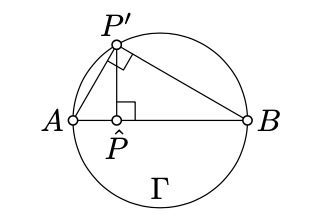Skip to main content
$$\newcommand{\id}{\mathrm{id}}$$ $$\newcommand{\Span}{\mathrm{span}}$$ $$\newcommand{\kernel}{\mathrm{null}\,}$$ $$\newcommand{\range}{\mathrm{range}\,}$$ $$\newcommand{\RealPart}{\mathrm{Re}}$$ $$\newcommand{\ImaginaryPart}{\mathrm{Im}}$$ $$\newcommand{\Argument}{\mathrm{Arg}}$$ $$\newcommand{\norm}{\| #1 \|}$$ $$\newcommand{\inner}{\langle #1, #2 \rangle}$$ $$\newcommand{\Span}{\mathrm{span}}$$

# 17.1: Special bijection on the h-plane

$$\newcommand{\vecs}{\overset { \rightharpoonup} {\mathbf{#1}} }$$ $$\newcommand{\vecd}{\overset{-\!-\!\rightharpoonup}{\vphantom{a}\smash {#1}}}$$$$\newcommand{\id}{\mathrm{id}}$$ $$\newcommand{\Span}{\mathrm{span}}$$ $$\newcommand{\kernel}{\mathrm{null}\,}$$ $$\newcommand{\range}{\mathrm{range}\,}$$ $$\newcommand{\RealPart}{\mathrm{Re}}$$ $$\newcommand{\ImaginaryPart}{\mathrm{Im}}$$ $$\newcommand{\Argument}{\mathrm{Arg}}$$ $$\newcommand{\norm}{\| #1 \|}$$ $$\newcommand{\inner}{\langle #1, #2 \rangle}$$ $$\newcommand{\Span}{\mathrm{span}}$$ $$\newcommand{\id}{\mathrm{id}}$$ $$\newcommand{\Span}{\mathrm{span}}$$ $$\newcommand{\kernel}{\mathrm{null}\,}$$ $$\newcommand{\range}{\mathrm{range}\,}$$ $$\newcommand{\RealPart}{\mathrm{Re}}$$ $$\newcommand{\ImaginaryPart}{\mathrm{Im}}$$ $$\newcommand{\Argument}{\mathrm{Arg}}$$ $$\newcommand{\norm}{\| #1 \|}$$ $$\newcommand{\inner}{\langle #1, #2 \rangle}$$ $$\newcommand{\Span}{\mathrm{span}}$$

Consider the conformal disc model with the absolute at the unit circle $$\Omega$$ centered at $$O$$. Choose a coordinate system $$(x,y)$$ on the plane with the origin at $$O$$, so the circle $$\Omega$$ is described by the equation $$x^2+y^2=1$$.The plane thru $$P, O$$, and $$S$$.

Let us think that our plane is the coordinate $$xy$$-plane in the Euclidean space; denote it by $$\Pi$$. Let $$\Sigma$$ be the unit sphere centered at $$O$$; it is described by the equation

$$x^2+y^2+z^2=1.$$

Set $$S=(0,0,-1)$$ and $$N=(0,0,1)$$; these are the south and north poles of $$\Sigma$$.

Consider stereographic projection $$\Pi\to\Sigma$$ from $$S$$; given point $$P\in\Pi$$ denote its image in $$\Sigma$$. Note that the h-plane is mapped to the north hemisphere; that is, to the set of points $$(x,y,z)$$ in $$\Sigma$$ described by the inequality $$z>0$$.

For a point $$P'\in \Sigma$$ consider its foot point $$\hat P$$ on $$\Pi$$; this is the closest point to $$P'$$.

Note that the composition $$P \leftrightarrow P' \leftrightarrow \hat{P}$$ of these two maps gives a bijection from the h-plane to itself. Further note that $$P=\hat{P}$$ if and only if $$P\in \Omega$$ or $$P=O$$.

Exercise $$\PageIndex{1}$$

Suppose that $$P\leftrightarrow \hat P$$ is the bijection described above. Assume that $$P$$ is a point of h-plane distinct from the center of absolute and $$Q$$ is its inverse in the absolute. Show that the midpoint of $$[PQ]$$ is the inversion of $$\hat{P}$$ in the absolute.

HintLet $$N, O, S, P, P'$$ and $$\hat{P}$$ be as on the diagram above.

Note that $$QQ = \dfrac{1}{x}$$ and therefore we need to show that $$O\hat{P} = 2/(x + \dfrac{1}{x})$$. To do this, show and use that $$\triangle SOP \sim \triangle SP'N \sim \triangle P'\hat{P}P$$ and $$2 \cdot SO = NS$$.

Lemma $$\PageIndex{1}$$

Let $$(PQ)_h$$ be an h-line with the ideal points $$A$$ and $$B$$. Then $$\hat P,\hat Q\in[AB]$$.

Moreover,

$\dfrac{A \hat{Q} \cdot B \hat{P}}{\hat{QB}\cdot \hat{P}A} = (\dfrac{AQ\cdot BP}{QB\cdot PA})^2.$

In particular, if $$A,P,Q,B$$ appear in the same order, then

$$PQ_h=\dfrac{1}{2} \cdot \ln \dfrac{A\hat{Q}\cdot B\hat{P}}{\hat{Q}B\cdot \hat{P}A}.$$

Proof

Consider the stereographic projection $$\Pi \to \Sigma$$ from the south pole $$S$$. Note that it fixes $$A$$ and $$B$$; denote by $$P'$$ and $$Q'$$ the images of $$P$$ and $$Q$$;

According to Theorem 16.3.1c,

$\dfrac{AQ\cdot BP}{QB\cdot PA}=\dfrac{AQ'\cdot BP'}{Q'B\cdot P'A}.$

By Theorem Theorem 16.3.1e, each circline in $$\Pi$$ that is perpendicular to $$\Omega$$ is mapped to a circle in $$\Sigma$$ that is still perpendicular to $$\Omega$$. It follows that the stereographic projection sends $$(PQ)_h$$ to the intersection of the north hemisphere of $$\Sigma$$ with a plane perpendicular to $$\Pi$$.

Suppose that $$\Lambda$$ denotes the plane; it contains the points $$A$$, $$B$$, $$P'$$, $$\hat P$$ and the circle $$\Gamma=\Sigma\cap\Lambda$$. (It also contains $$Q'$$ and $$\hat{Q}$$ but we will not use these points for a while.)The plane $$\Lambda$$.

Note that

• $$A,B,P'\in\Gamma$$,
• $$[AB]$$ is a diameter of $$\Gamma$$,
• $$(AB)=\Pi\cap\Lambda$$,
• $$\hat{P} \in [AB]$$
• $$(P'\hat{P} \perp (AB).$$

Since $$[AB]$$ is the diameter of $$\Gamma$$, by Corollary 9.8, the angle $$AP'B$$ is right. Hence $$\triangle A\hat PP' \sim \triangle AP'B \sim \triangle P'\hat PB$$. In particular

$$\dfrac{AP'}{BP'}=\dfrac{A \hat{P}}{P'\hat{P}}=\dfrac{P'\hat{P}}{B\hat{P}}.$$

Therefore

$\dfrac{A\hat{P}}{B\hat{P}}=(\dfrac{AP'}{BP'})^2.$

The same way we get that

$\dfrac{A\hat{Q}}{B\hat{Q}}=(\dfrac{AQ'}{BQ'})^2.$

Finally, note that 17.1.2+17.1.3+17.1.4 imply 17.1.1

The last statement follows from 17.1.1 and the definition of h-distance. Indeed,

\begin{aligned} PQ_h&:=\ln\frac{A Q\cdot B P}{QB\cdot PA}= \\ &=\ln\left(\frac{A \hat Q\cdot B \hat P}{\hat QB\cdot \hat PA}\right)^{\frac12}= \\ &=\tfrac12\cdot\ln\frac{A \hat Q\cdot B \hat P}{\hat QB\cdot \hat PA}.\end{aligned}

Exercise $$\PageIndex{2}$$

Let $$\Gamma_1$$, $$\Gamma_2$$, and $$\Gamma_3$$ be three circles perpendicular to the circle $$\Omega$$. Let $$[A_1B_1]$$, $$[A_2B_2]$$, and $$[A_3B_3]$$ denote the common chords of $$\Omega$$ and $$\Gamma_1$$, $$\Gamma_2$$, $$\Gamma_3$$ respectively. Show that the chords $$[A_1B_1]$$, $$[A_2B_2]$$, and $$[A_3B_3]$$ intersect at one point inside $$\Omega$$ if and only if $$\Gamma_1$$, $$\Gamma_2$$, and $$\Gamma_3$$ intersect at two points.Hint

Consider the bijection $$P \leftrightarrow \hat{P}$$ of the h-plane with absolute $$\Omega$$. Note that $$\hat{P} \in [A_iB_i]$$ if and only if $$P \in \Gamma_i$$.